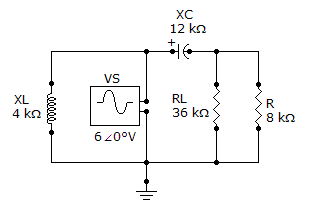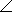# Electrical Engineering - Circuit Theorems in AC Analysis - Discussion

### Discussion :: Circuit Theorems in AC Analysis - General Questions (Q.No.4)

4.

For the circuit shown, determine ZTH for the portion of the circuit external to RL.[A]. 66.7–33.7° k[B]. 6.67–333.7° k[C]. 14.4–56.3° k[D]. 1.44–33.7° kExplanation:

No answer description available for this question.

 Nikhil Jain said: (Jun 29, 2014) Parallel combination of j12 kohm and 8 kohm will be the answer.

 Edmond Chiu said: (Apr 24, 2017) Answer B should be 6.67 Kohm angle -33.7 degree.

 Sakti Saurav said: (Mar 5, 2021) z = 8+j 12 Magnitude of z = √ (64+144). z = 14.42.